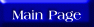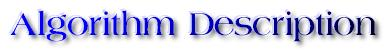Quicksort is based on the divide and conquer approach and inspite of its slow worst case running time, it is often the best practical choice for sorting because it is remarkably efficient on the average. The following code describes the algorithm:

QuickSort(A, p, r){
if p < r
then q <- Partition(A, p, r)
QuickSort(A, p, q)
QuickSort(A, q+1, r)
}

To sort an entire array A, the initial call is QuickSort(A, 1, length[A]).

Partition(A, p, r){
x <- A[p]
i <- p - 1
j <- r + 1
while TRUE
do repeat j <- j - 1
until A[j] <= x
repeat i <- i + 1
until A[i] >= x
if i < j
then exchange A[i] <-> A[j]
else return j
}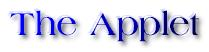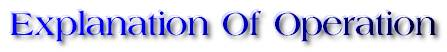The algorithm starts by first performing a scan of the entire list, as indicated by the two black items at the two ends of the list. It attempts then to partition the list. The manner in which this partitioning is done is: First, the pivot element is considered to be the first element of the (sub)list being scanned, i.e., element 0. The high-pointer is shown in green and the low-pointer in blue. Scanning of the (sub)list consists of decrementing the high-pointer and incrementing the low-pointer until A[low-pointer]>= A[pivot] >= A[high-pointer]. As the scan of the (sub)list proceeds, the magnitudes of the list elements are compared with the magnitude of the pivot. First the high-pointer is decremented till it meets an element that is less than or equal to the pivot. Then the low-pointer is incremented till it meets an element greater than equal to the pivot. If the low-pointer is less than the high-pointer, then the elements pointed to by these are swapped else the element pointed by the high-pointer becomes the pivot. Conceptually, the partitioning procedure performs a simple function: it puts elemnts smaller than the pivot into the bottom region of the array and elements larger than the pivot into the top region. Now, the same partitioning approach can be recursively applied to the smaller sublist and also to the larger sublist obtained as a result of the partitioning. Repeated applications of this approach to all sublists generated results in a sorted list.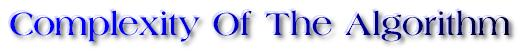Quicksort works by partitioning the list into two parts. The first partitioning of the N elements in list positions 0 to N-1, compares and swaps them to produce a pivotal index j such that the elements in positions 0 to j-1 are less than or equal to the pivot which is now in the jth position and those elements in positions j+1 to N-1 are greater. This is accomplished in O(N) time.
The first partition produces two sublists such that sorting of each of them results in the sorting of the original list. The partitioning technique is applied recursively to each half until the halves reduce to single-element or empty lists.
Assuming that the list breaks into two halves of equal size, then we have two lists of size N/2 to sort. Each of these has to be partitioned which takes (N/2) + (N/2) = N comparisions. Continuing the same assumption, there will be atmost log(N) splits. This results in the best case time estimate of O(N*log(N)) for quicksort.
In the worst case, the partitioning routine produces one region with (N-1) elements and one with only one element (this happens when the list to be sorted is already sorted). This gives a worst case time of O(N^2). However, quicksort takes O(N*log(N)) in the average case.2